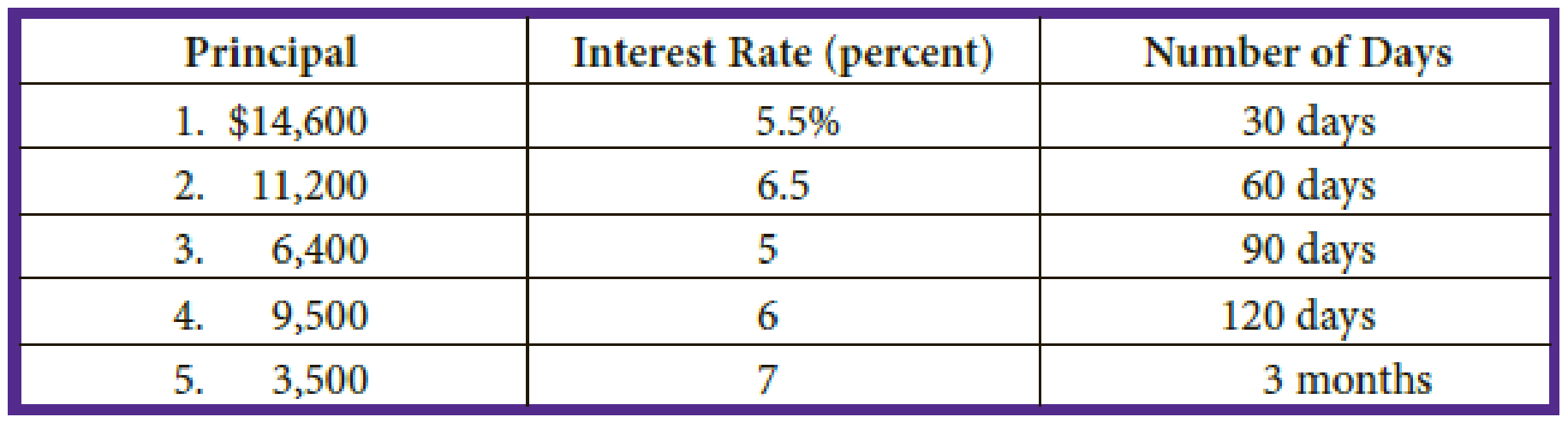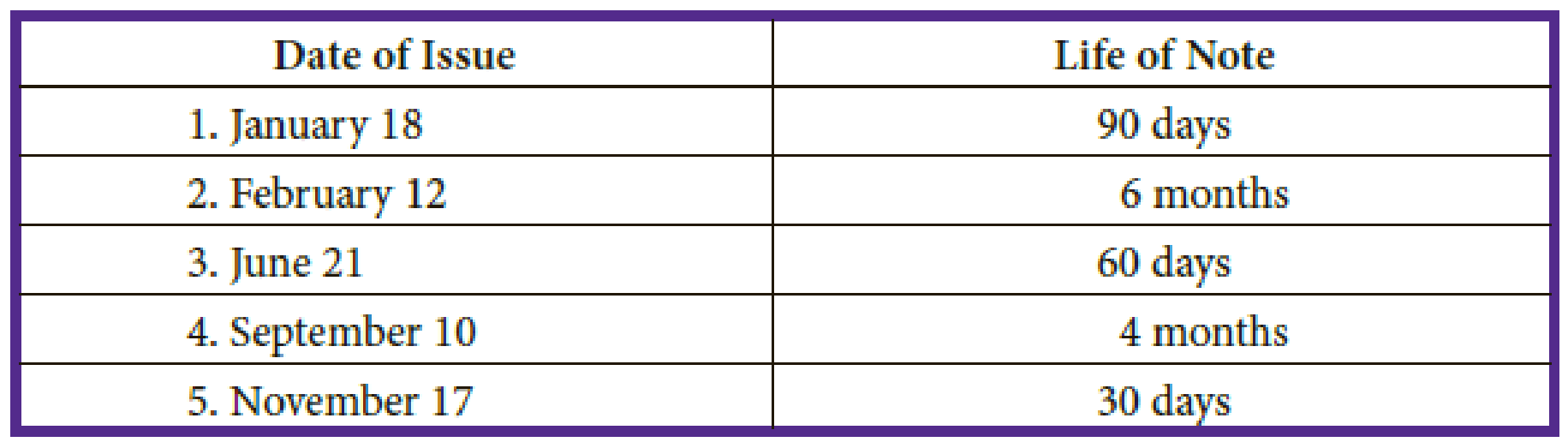# Part A: Calculate the interest on the following notes: Part B: Determine the maturity dates on the following notes: Check Figure 1. Interest, $66.92BuyFind ### College Accounting (Book Only): A ... 13th Edition Scott + 1 other Publisher: South-Western College Pub ISBN: 9781337280570BuyFind ### College Accounting (Book Only): A ... 13th Edition Scott + 1 other Publisher: South-Western College Pub ISBN: 9781337280570 #### Solutions Chapter Section Chapter D, Problem 1P Textbook Problem ## Part A: Calculate the interest on the following notes:Part B: Determine the maturity dates on the following notes:Check Figure1. Interest,$66.92

Expert Solution

A.

To determine

Compute the interest for the given notes.

### Explanation of Solution

Interest: The money charged by the lender for using lender’s funds is referred to as interest. So, interest is an expense for the borrower and revenue for the lender. The interest is generally, computed as a percentage of the money borrowed.

Compute the interest for the given notes.

 Principal × Rate of Interest × Time of Note = Interest 1. $14,600 × 5.5% × 30 days ÷ 360 days =$66.92 2. 11,200 × 6.5% × 60 days ÷ 360 days = 121.33 3. 6,400 × 5.0% × 90 days ÷ 360 days = 80.00 4. 9,500 × 6.0% × 120 days ÷ 360 days = 190.00 5. 3,500 × 7.0% × 3 months ÷ 12 months = 61.25

Table (1)

Expert Solution

B.

To determine

Compute the maturity dates.

### Explanation of Solution

Maturity date: The date on which the borrower should pay the principal amount of loan, or bond, is referred to as maturity date.

Compute the maturity date for the note issued on January 18 with the life of note for 90 days.

 Description Count Number of days left in January (From 18th to 31st) 13 days Number of days in February 28 days Number of days in March 31 days 72 days Number of days required in April to total 90 days (90 days–72 days) 18 days Total days of the term 90 days

Table (2)

Hence, the maturity date is 18th April.

Compute the maturity date for the note issued on February 12 with the life of note for 6 months.

 Description Count Number of months from February 12 to March 12 1 month Number of months from March 12 to April 12 1 month Number of months from April 12 to May 12 1 month Number of months from May 12 to June 12 1 month Number of months from June 12 to July 12 1 month Number of months from July 12 to August 12 1 month Total months of the term 6 months

Table (3)

Hence, the maturity date is 12th August.

Compute the maturity date for the note issued on June 21 with the life of note for 60 days.

 Description Count Number of days left in June (From 21st to 30th) 9 days Number of days in July 31 days 40 days Number of days required in August to total 60 days (60 days–40 days) 20 days Total days of the term 60 days

Table (4)

Hence, the maturity date is 20th August.

Compute the maturity date for the note issued on September 10 with the life of note for 4 months.

 Description Count Number of months from September 10 to October 10 1 month Number of months from October 10to November 10 1 month Number of months from November 10 to December 10 1 month Number of months from December 10 to January 10 1 month Total months of the term 4 months

Table (5)

Hence, the maturity date is 10th January.

Compute the maturity date for the note issued on November 17 with the life of note for 30 days.

 Description Count Number of days left in November (From 17th to 30th) 13 days Number of days required in December to total 30 days (30 days–13 days) 17 days Total days of the term 30 days

Table (6)

Hence, the maturity date is 17th December.

### Want to see more full solutions like this?

Subscribe now to access step-by-step solutions to millions of textbook problems written by subject matter experts!

Get Solutions

### Want to see more full solutions like this?

Subscribe now to access step-by-step solutions to millions of textbook problems written by subject matter experts!

Get Solutions

Find more solutions based on key concepts
Show solutions
What is an REA diagram?

Accounting Information Systems

Why are there so many laws relating to HRM practices?

Foundations of Business (MindTap Course List)

Why do economists oppose policies that restrict trade among nations?

Principles of Macroeconomics (MindTap Course List)

PURCHASING POWER PARITY In the spot market, 12.8 Mexican pesos can bo exchanged for 1 U.S. dollar. A compact di...

Fundamentals of Financial Management, Concise Edition (with Thomson ONE - Business School Edition, 1 term (6 months) Printed Access Card) (MindTap Course List)